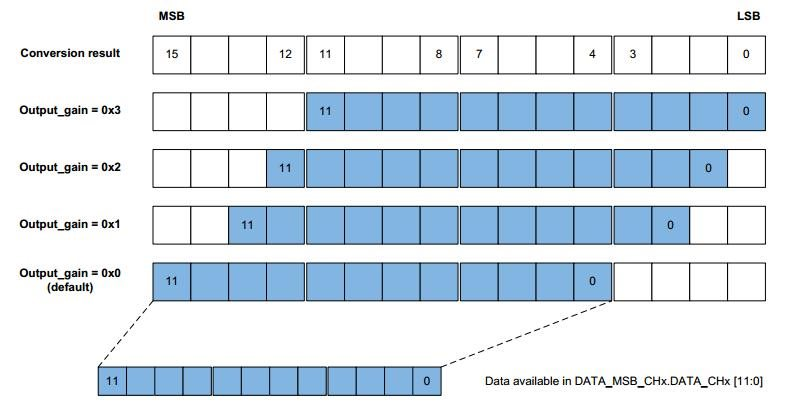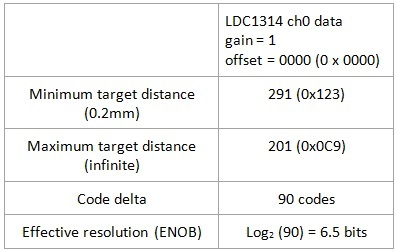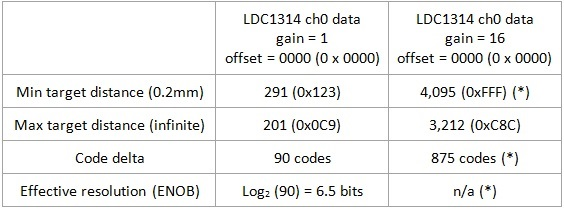# Inductive sensing: Improve the ENOB of a multichannel LDC by 4 bits in 3 simple steps

In a recent inductive sensing blog post, I introduced the new new multichannel LDCs: the 12-bit LDC1312 and LDC1314 and the 28-bit LDC1612 and LDC1614.

There are scenarios in which you might want to use the 12-bit LDC1312 or LDC1314 due to higher sample rates or lower cost, when the resolution of the 12-bit LDC isn’t quite high enough for your particular system needs, for example in this 1-degree dial. In this case, you can use the gain and offset registers to improve the effective number of bits (ENOB) by up to 4 bits. Measurement timing is unaffected by using gain and offset.

The gain/offset feature works because the LDC1312 and LDC1314 have an internal 16-bit data converter, but they only display 12 of those bits in the data registers: DATA_CH0, DATA_CH1, DATA_CH2 and DATA_CH3. By default, the gain feature is disabled and the DATA registers display the 12 most significant bits (MSBs) of the 16-bit word. However, it is possible to shift the data output; see Figure 1.Figure 1: Conversion data output gain

Employing the gain of 4x, 8x or 16x causes a 2-bit, 3-bit or 4-bit data shift, which is equivalent to increasing the maximum effective number of bits by 2 bits, 3 bits or 4 bits, respectively.

Let’s use a simple example to illustrate how gain and offset work. The example uses an LDC1314 evaluation module. My target is a U.S. quarter that moves between a 0.2mm target distance and an infinite target distance.

Here’s how to optimize the resolution in three simple steps:

1. Determine system boundaries. When moving between the minimum target distance (0.2mm) and maximum target distance (infinite), I measure the following (Table 1):Table 1: ENOB before applying gain

Using the gain feature discards the MSBs; therefore, it’s important to ensure that the maximum output scale does not go below zero or above the full scale of the new data output. The datasheet shows that the maximum output range must be:

• ≤ 100% of full scale with gain = 1x
• ≤ 25% of full scale with gain = 4x
• ≤ 12.5% of full scale with gain = 8x
• ≤ 6.25% of full scale with gain = 16x

2. Apply gain. The full-scale data word is 212-1 = 4,095. My example shows a delta of 90 codes between the minimum and maximum target positions, which is only 2.2% of full scale. I can therefore comfortably use the maximum gain setting of 16x. Under this condition, I measure the following (Table 2):((*) signal is clipped

Table 2: ENOB after applying gain

My code delta has improved greatly, but the data output at the minimum target distance clips at the full scale of my new data word.

3. Subtract offset. While my system only uses 2.2% of full scale, it crosses the full-scale boundary, which results in a loss of information. To fix this issue, I can use the offset register to subtract a fixed offset from the data output. The maximum target distance output code is 3,212, so I can easily subtract 2,000 codes.

(*) signal is clipped

Table 3: ENOB after applying gain and offset

With a gain of 16x and an offset of -2,000 codes, the LDC now records data between 1,212 and 2,670, as shown in Table 3. This is well within the output-code limits of 0 to 4,095. The code delta is 1,458 codes, which is a 4-bit improvement over the default case with a gain of 1.

Figure 2 shows the operating output range for this example without gain, with a gain of 16, and with a gain of 16 and an offset of -2,000.

Figure 2: Conversion signal range increases after applying gain and offset

What if the resolution is still insufficient?

In this simple example, the effective resolution improved by 4 bits (from 6.5 bits to 10.5 bits) without any impact on timing or power consumption. If this effective resolution is still insufficient for your system, consider using one of our 28-bit multichannel devices, the LDC1612 or LDC1614.You can read more about the resolution benefits of the the LDC1612 and LDC1614 here.

Leave a note below and let me know future topics you’d like for me to discuss about multichannel LDCs.